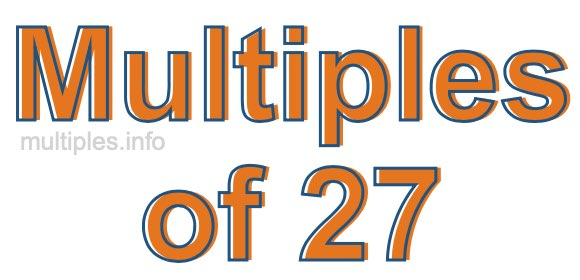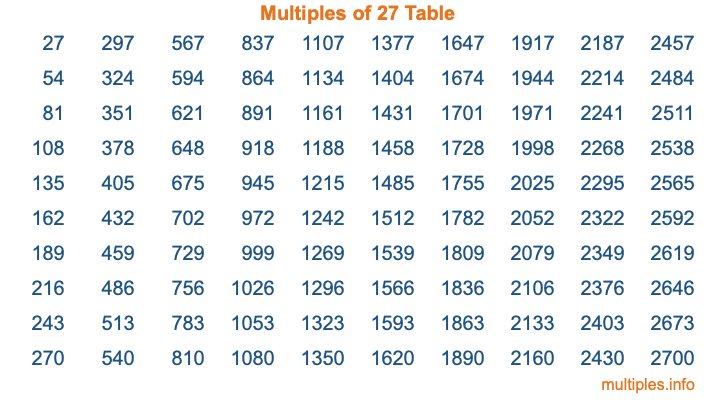Multiples of 27Welcome to the Multiples of 27 page. Here we will first teach you everything you will ever need to know about the multiples of 27, and then give you a study guide summary of everything we taught you to make sure you remember it all. Use this page to look up facts and learn information about the multiples of 27. This page will make you a multiples of twenty-seven expert!

Definition of Multiples of 27
Multiples of 27 are all the numbers that when divided by 27 equal an integer. Each of the multiples of 27 are called a multiple. A multiple of 27 is created by multiplying 27 by an integer.

Therefore, to create a list of multiples of 27, you start with 1 multiplied by 27, then 2 multiplied by 27, then 3 multiplied by 27, and so on for as long as you want. Thus, the list of the first five multiples of 27 is 27, 54, 81, 108, and 135. To see a larger list of multiples of 27, see the printable image of Multiples of 27 further down on this page. We also have a category where you can choose any nth multiple of 27.

Multiples of 27 Checker
The Multiples of 27 Checker below checks to see if any number of your choice is a multiple of 27. In other words, it checks to see if there is any number (integer) that when multiplied by 27 will equal your number. To do that, we divide your number by 27. If the the quotient is an integer, then your number is a multiple of 27.

Is  a multiple of 27?

Least Common Multiple of 27 and ...
A Least Common Multiple (LCM) is the lowest multiple that two or more numbers have in common. This is also called the smallest common multiple or lowest common multiple and is useful to know when you are adding our subtracting fractions. Enter one or more numbers below (27 is already entered) to find the LCM.

Check out our LCM Calculator if you need more details about the Least Common Multiple or if you need the LCM for different numbers for adding and subtraction fractions.

nth Multiple of 27
As we stated above, 27 is the first multiple of 27, 54 is the second multiple of 27, 81 is the third multiple of 27, and so on. Enter a number below to find the nth multiple of 27.

th multiple of 27

Multiples of 27 vs Factors of 27
27 is a multiple of 27 and a factor of 27, but that is where the similarities end. All postive multiples of 27 are 27 or greater than 27. All positive factors of 27 are 27 or less than 27.

Below is the beginning list of multiples of 27 and the factors of 27 so you can compare:

Multiples of 27: 27, 54, 81, 108, 135, etc.

Factors of 27: 1, 3, 9, 27

As you can see, the multiples of 27 are all the numbers that you can divide by 27 to get a whole number. The factors of 27, on the other hand, are all the whole numbers that you can multiply by another whole number to get 27.

It's also interesting to note that if a number (x) is a factor of 27, then 27 will also be a multiple of that number (x).

Multiples of 27 vs Divisors of 27
The divisors of 27 are all the integers that 27 can be divided by evenly. Below is a list of the divisors of 27.

Divisors of 27: 1, 3, 9, 27

The interesting thing to note here is that if you take any multiple of 27 and divide it by a divisor of 27, you will see that the quotient is an integer.

Multiples of 27 Table
Below is an image of the first 100 multiples of 27 in a table. The table is in chronological order, column by column. The first column has the first ten multiples of 27, the second column has the next ten multiples of 27, and so on.The Multiples of 27 Table is also referred to as the 27 Times Table or Times Table of 27. You are welcome to print out our table for your studies.

Negative Multiples of 27
Although not often discussed or needed in math, it is worth mentioning that you can make a list of negative multiples of 27 by multiplying 27 by -1, then by -2, then by -3, and so on, to get the following list of negative multiples of 27:

-27, -54, -81, -108, -135, etc.

Multiples of 27 Summary
Below is a summary of important Multiples of 27 facts that we have discussed on this page. To retain the knowledge on this page, we recommend that you read through the summary and explain to yourself or a study partner why they hold true.

There are an infinite number of multiples of 27.

A multiple of 27 divided by 27 will equal a whole number.

27 divided by a factor of 27 equals a divisor of 27.

The nth multiple of 27 is n times 27.

The largest factor of 27 is equal to the first positive multiple of 27.

27 is a multiple of every factor of 27.

27 is a multiple of 27.

A multiple of 27 divided by a divisor of 27 equals an integer.

27 divided by a divisor of 27 equals a factor of 27.

Any integer times 27 will equal a multiple of 27.

Multiples of a Number
Here you can get the multiples of another number, all with the same attention to detail as we did for multiples of 27 on this page.

Multiples of
Multiples of 28
Did you find our page about multiples of twenty-seven educational? Do you want more knowledge? Check out the multiples of the next number on our list!